Mobile QR Code1. (Department of Electrical and Electronics Engineering, Konkuk University, Seoul 05029, Korea)
2. (School of Electronic and Electrical Engineering, Sungkyunkwan University, 16419, Suwon, Korea)

Gate-all-around FET, process design kit, parasitic extraction, benchmark, interconnect

## I. INTRODUCTION

The front-end-off-line (FEOL) transistor scaling down technology through ultra-fine processes has led to the continuous development of semiconductor technology. In order to continue the development of technology through continuous scaling down, the two-dimensional planar gate-based metal-oxide-semiconductor field-effect transistor (MOSFET) structure has evolved into a three-dimensional structure that increases gate controllability such as Fin-FET and GAA-FET to mitigate short-channel effects [1-6]. As the process becomes scaled down, the impact of resistance and capacitance(RC) components parasitic on circuits on middle-of-line (MOL) and back-end-of-line (BEOL), including local interconnects, has become as important as FEOL [7-9]. Since RC inevitably increase when wire is miniaturized, a change in wire material that can reduce wire RC has been required to achieve continuous scaling down. The most notable change is occurring in the lowest metal layer (M1) and the local interconnect (or it called as M0 layer) between the source/drain/gate contact and M1. Cu, a wire metal material introduced after the 130 nm process node, is required to be transited to another wire material at the scaled down node, so research results on many materials have been reported [10-14]. The line width of Cu in 7 nm node (15nm~20nm) is smaller than the MFP of Cu (39.9nm). This increases the surface scattering of electrons. Therefore, Cu is unsuitable for use as an interconnect below the 7 nm node . Materials that have been proposed to replace Cu include Co, Ru, and DMLG. Co has higher EM reliability and lower Line-R than Cu. Cu requires a thicker barrier, while Co does not . Despite having a larger bulk resistivity than Cu, Ru has a low Line-R due to the large-grained Ru lines and the absence of a high aspect ratio barrier like Cu . DMLG with proper doping is shown to have the resistivity and performance of sub-20 nm Cu theoretically. Experiments have since been reported to improve reliability and performance . As far as we know, more analysis results are needed because the metal materials for the next generation of wire have not been determined.

In order to investigate the influence of such MOL and BEOL, layout-based circuit level evaluation is necessary. Recently, the results of investigating the effect of wire on circuit characteristics were reported by developing a Process-Design-Kit (PDK) including a wire model and a transistor model that can describe the characteristics of MOL, BEOL, and FEOL [17-20]. Furthermore, research result has been reported on the critical dimension of the wire process that allows certain metal materials to satisfy the target wire RC at a specific process node . In our previous work , it is reported that the effects of wire processes consisting of W and Cu on circuits at 3 nm technology nodes with mNS-FET. In addition, the impact of air spacer technology with low wire capacitance on the corresponding technology node was reported. However, there are insufficient works on circuit-level analysis for a variety of metal material options that are considered the future technology at reduced line widths of the latest process node.

In this work, the effects of 5 wire metal materials (Cu, W, Co, Ru, and DMLG) on circuit level power and performance at 3 nm process node were benchmarked. Then we simulate the PPA properties according to the MOL stack structure and tangent ratio, which is a distinctly different research direction from the previous paper. For this purpose, the wire resistance values that vary in the dimension of various wire metal materials reported by Kunjesh Agashiwala et. al. in  through atomic-level simulation and measurement of fabricated hardware were utilized. In addition, the effect of each MOL and BEOL component on the circuit characteristics was observed using the segmentation technique. We believe that our findings will help select wire materials for low-power or/and high-performance applications. The next chapter II describes the simulation analysis environment used in the work, and shows the analysis results at the 3 nm circuit level for various wire metal options. And finally, we will conclude in Chapter III.

## II. PATH-FINDING PROCESS-DESIGN-KIT (PDK) SETUP AND LOGIC INTEGRATED CIRCUIT ANALYSIS RESULTS

### 1. Path-finding PDK : FEOL Model

In this work, a mNS-FET with the 3 nm process node dimension was used as a FEOL device. As shown in Fig. 1, it is a structure in which three lateral nanosheets are vertically stacked, and the main dimension is the 3 nm process node set value defined in IRDS$^{\mathrm{TM}}$2021 edition , and the used values are summarized in Table 1. The electrical characteristics were obtained by simulation using Sentaurus$^{\mathrm{TM}}$, a TCAD software of Synopsys. Used TCAD was calibrated with measurements of the fabricated 7 nm mNS-FET device . In addition, the electrical I-V and C-V characteristic curves obtained from the TCAD simulation are described using the BSIM-CMG compact model, and a BSIM-CMG model library  was developed by extracting the BSIM-CMG model parameters to describe it. This enables the simulation of dynamic circuit characteristics. As such, the BSIM-CMG model library for the 3 nm mNS-FET obtained from TCAD was targeted to improve speed and power by +20% and -30%, respectively, at the same supply voltage (V$_{\mathrm{DD}}$=0.7 V) based on the reported 5 nm target  to satisfy the circuit operation target of the 3nm process node. Through this process, the final 3 nm mNS-FET model library was developed. This process is illustrated in Fig. 2(a)-(c). Further details on the carrier transport model used in TCAD simulation and BSIM-CMG model library targeting can be found in our previous work . The impact of contact resistivity value on the electrical characteristics of FEOL devices in sub-nm technology nodes is significant and must be taken into account. Therefore, this paper considers the contact resistivity value when calculating the electrical characteristics of the FEOL device through TCAD simulation, resulting in the inclusion of source/drain contact resistance in the extracted BSIM-CMG model library. We used the contact resistivity value of 1\times10-9 ${\Omega}$·cm, which is suggested as the target value for the 3 nm technology node in .

##### Fig. 1. 3D structure and cross sectional area of mNS-FET.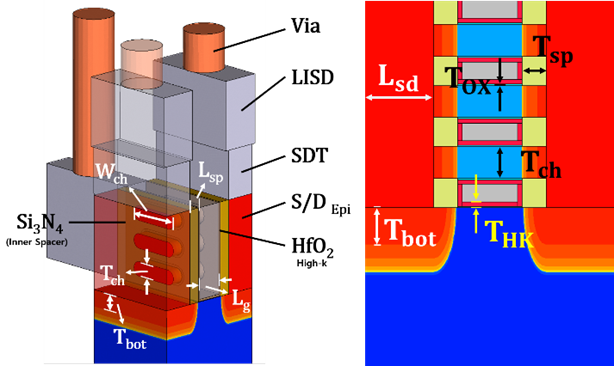##### Fig. 2.(a) An overall flow chart of mNS-FET-based element and circuit analysis; (b) Calibration result of transport model parameter using TCAD software; (c) FO3 INV RO Simulation Results for IOFF Targeting and 3 nm Technology Node.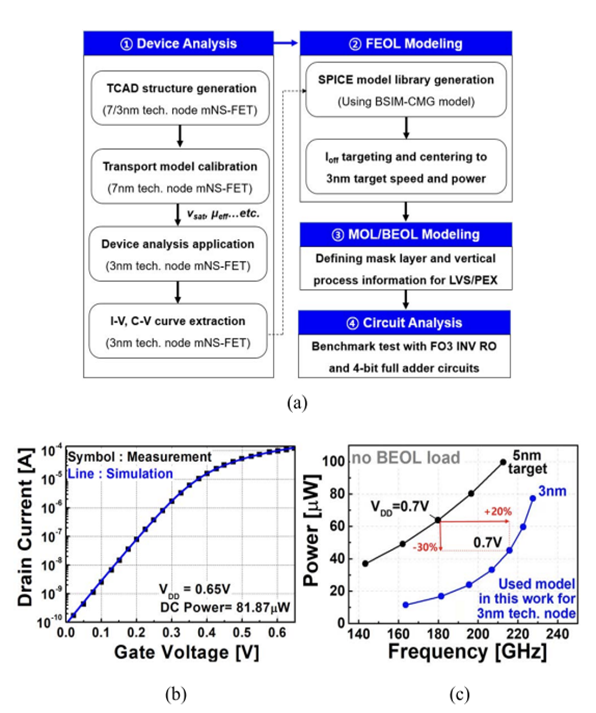##### Table 1. mNS-FET key parameter for 3 nm technology node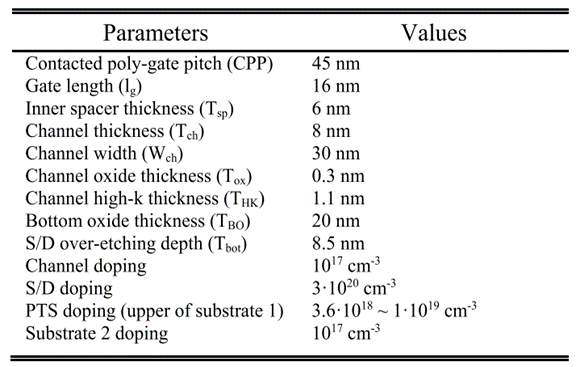### 2. Path-finding PDK : MOL, BEOL Model

The wire metal materials considered in this work have a total of 5 types (W, Cu, Co, Ru, DMLG), each with different electrical characteristics. In the case of the currently widely used Cu/W wire material, a barrier metal is required, and as the wire dimension decreases, it is difficult to scale together, which increases the wire resistance, and thus the difficulty of continuous application is expected. However, unlike conventional Cu, Co can be processed without barriers . The resistance of barrierless Co is greater than that of conventional Cu, but for an aspect ratio of 2 (AR 2), below 16 nm CD has less resistance than Cu when using barrierless Co. Barrierless Ru is also compatible with CMOS processes [14-16]. Ruthenium is a material being used and researched in fabs and is one of the next generation IRDS  metal interconnects. Currently, the dual damascene process, which is widely used, has intensified issues such as non-linear resistivity size effect problem due to electro-migration, deterioration problem due to self-heating, and void in trench and via region in metal fill process as the dimension becomes smaller . On the other hand, the single damascene process resolves the problem of misalignment between wire metal and via, so the cause of self-heating and current crowding can be decreased, and the vertical length can be reduced due to edge contact scheme, so it can have lower resistance. Recently, in [23,26], it was reported that DMLG can be implemented through a single damascene process, does not require a barrier such as Co/Ru, and has better conductivity in a smaller aspect ratio (AR) than Co/Ru due to reduced surface and particle boundary scattering. DMLGs are also highly reliable for self-heating (SH) and electron migration (EM). DMLG is produced by depositing Ni on SiO2 and then applying pressure to the graphene power to grow graphene by diffusing carbon through Ni at the Ni/SiO2 interface. It was assumed that multi-walled CNTs, which always show metallic properties, were applied to vias implemented with DMLG.

For circuit simulation considering the wire resistance in MOL and BEOL with wire metal materials of W, Cu, Co, Ru, and DMLG, it is necessary to model the (sheet) resistance (or resistivity) in via and wire metal with these materials and configure a distributed RC network in the interconnect region from the layout generated by the circuit designer. For this purpose, vertical process information for 3 nm integrated circuit is as follows. The MOL region interconnect takes the form of stacking contact vias and local interconnects from the source/drain/gate contacts of the FEOL device to M1. In this work, contact via and local interconnect are referred to as source-drain trench (SDT) and local-interconnect source-drain (LISD), respectively. Fig. 3 depicts the inter-layer dielectric (ILD) and inter-metal dielectric (IMD) as well as the overall dimensions and structures of the MOL and BEOL regions. The relative dielectric constant values of each ILD and IMD are shown in the table in Fig. 3. This satisfies C$_{\mathrm{W}}$ = 209 aF/${\mu}$m, the target value of interconnect capacitance (C$_{\mathrm{W}}$) per unit length of the 3 nm process node in IRDS$^{\mathrm{TM}}$2021 edition .

As such, the capacitance value in the wire was fixed to the 3 nm process node value presented by IRDS$^{\mathrm{TM}}$2021 edition, and the effect of five wire metal materials (Cu, W, Co, Ru, and DMLG) on the circuit-level characteristics in the 3 nm process node was analyzed. To this end, various wire metal materials reported by Kunjesh Agashiwala through atomic-level simulation and manufactured hardware measurement in  utilized the resistance values in the metal layer and via with considering variable dimension, which are summarized in Fig. 4(a) and (b). Considering the nanosheet width and source/drain region length in the mNS-FET used in this work, the dimension of SDT was 17 nm x 30 nm, so the via resistance value when the via width was 17 nm was referred to in Fig. 4(b), and then scaled according to the dimension. Therefore, SDT resistance values for each material are W 30 ${\Omega}$, Cu 12 ${\Omega}$, Co 23 ${\Omega}$, Ru 7 ${\Omega}$, and CNT 57 ${\Omega}$. Since the dimension of V0 is 8 nm x 8 nm, the fV0 resistance values obtained using the value when the via width is 8 nm in Fig. 4(b) are W 230 ${\Omega}$, Cu 110 ${\Omega}$, Co 120 ${\Omega}$, Ru 45 ${\Omega}$, and CNT 340 ${\Omega}$. In the case of LISD, since it is a local interconnect, the total resistance value was targeted in consideration of the wire resistance (R$_{\mathrm{W}}$) per unit length from Fig. 4(a). The LISD resistance values for each material are W 22 ${\Omega}$, Cu 4 ${\Omega}$, Co 4 ${\Omega}$, Ru 8~${\Omega}$, and DMLG 3~${\Omega}$. Since metal1 (M1) also corresponds to an interconnect, the R$_{\mathrm{W}}$ value in Fig. 4 was used. The R$_{\mathrm{W}}$ of M1 for each material are W 200 ${\Omega}$/㎛, Cu 500 ${\Omega}$/㎛, Co 400 ${\Omega}$/㎛, Ru 580 ${\Omega}$/㎛, and DMLG 220 ${\Omega}$/㎛. As shown in Fig. 5, a mask for each region was defined using a layout editor (Silvaco's EXPERT), and electronic design automation (EDA) software (Synopsys's StarRC, IC Validator) was applied to each mask layer and used during circuit analysis. This analysis environment makes it possible to segment and analyze the effect of each RC component of MOL and BEOL on circuit behaviors.

##### Fig. 3. The cross-sectional area of the MOL and BEOL regions and the key parameters##### Fig. 4. (a) Resistance per unit length for wire width of Cu, W with 2 nm barrier layer and Co, Ru, intercalation-doped MLG without barrier layer. (AR=2); (b) Via resistance for the via width of Cu, W with 2 nm barrier layer and Co, Ru, and CNT without barrier layer. (AR=2) The width and length are the same square shape.##### Fig. 5. Circuit diagram of FO3 INV RO with INV unit cell layout. The RC network of MOL/BEOL wiring in Layout is automatically extracted through path-finding PDK.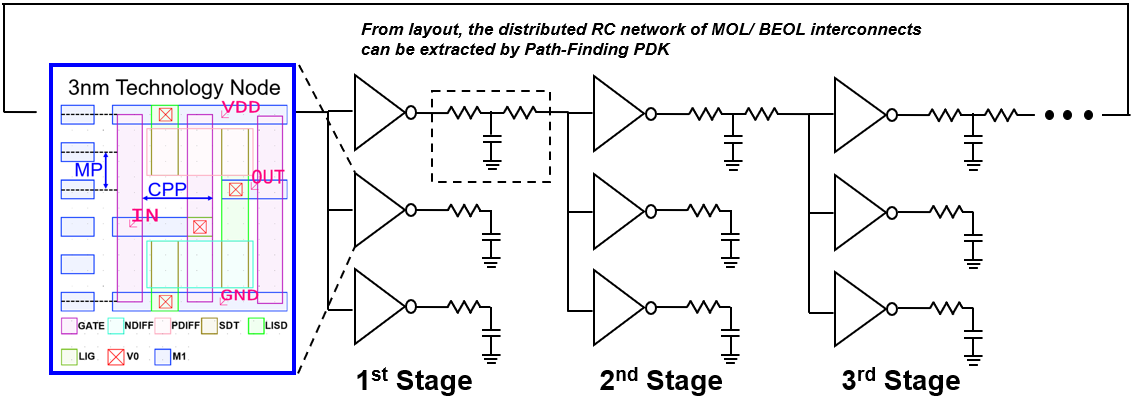### 3. Logic Integrated Circuit Analysis with Various Wire Metal Options

As described above, the post-layout simulation of the INV RO circuit was conducted using 5 path-finding PDKs manufactured for each wire metal material. By analyzing the power and performance characteristics of INV RO circuit during logic operation, it was confirmed how the circuit characteristics change for each wire metal material. Power represents the active power consumption, and performance represents the operating frequency where it means the reciprocal value of delay standardized by the number of INV stages. Fig. 5 shows the fan-out 3(FO3) INV 9-stages RO (RO9), and the layout of the INV unit cell was composed of CPP=45 nm, MP=24 nm and 5 tracks to suit the 3 nm process node. In addition, the change in circuit characteristics by MOL/BEOL was confirmed by changing the wire length and FO number between stages of INV RO.

Fig. 6(a)-(e) shows the results of observing the change in circuit characteristics for each wire metal material used in MOL/BEOL when MOL resistance (R$_{\mathrm{MOL}}$), MOL capacitance (C$_{\mathrm{MOL}}$), BEOL resistance (R$_{\mathrm{BEOL}}$), and BEOL capacitance (C$_{\mathrm{BEOL}}$) are sequentially added in the intrinsic mNS-FET. The circuit used at this analysis was FO1 INV RO9, and the wire length between stages was based on 25CPP (=1125 nm). Based on the supply voltage (VDD)=0.7 V, W, Cu, Co, Ru, and DMLG all show the same circuit characteristics in FEOL where the MOL/BEOL region has been removed. The analysis results below follow the order of W, Cu, Co, Ru, and DMLG. When R$_{\mathrm{MOL}}$ is added, the speed decreases by -8%, -5%, -3%, and -11%, respectively, and the power decreases by -8%, -4%, -2%, and -10%, respectively. The speed reduction by R$_{\mathrm{BEOL}}$ is -9%, -3%, -1%, -3%, and -2%, respectively, and the power reduction is -18%, -7%, -5%, -7%, and -4%, respectively. In the case of circuit characteristics that use W-based wire metal, the speed is the slowest to decrease by -78% compared to the intrinsic property, but the power is the lowest due to a decrease of -26%. This is because the power consumption of a circuit is proportional to the product of the operating frequency, capacitance, and supply voltage of the circuit, so the decrease in speed appears to be a decrease in power consumption. Conversely, Co and Ru are the fastest in terms of speed, with -72% decrease compared to the intrinsic property, but power is high at -8% decrease compared to the intrinsic property. Considering both the speed and the power, it can be seen that using Cu, which is mainly used for current wire metal, or DMLG, which is in the spotlight as a next-generation wire metal, is the most effective in improving circuit characteristics. Fig. 6(f) shows the proportion of effective resistance that affects delay by each component. In the case of R$_{\mathrm{MOL}}$, DMLG has the greatest impact on the effective resistance with 9.5%, which is the largest than other options, and the speed by adding R$_{\mathrm{MOL}}$ is also reduced by -11%, which is larger than the other wire metal options. In the case of R$_{\mathrm{BEOL}}$, W has the greatest impact on the effective resistance with 19.9%, which is the largest than other options, and the speed by adding R$_{\mathrm{BEOL}}$ is also reduced by -9%, which is larger than the other options.

Fig. 7(a)-(e) shows the change in circuit characteristics according to the BEOL load by changing the wire length (L$_{\mathrm{WIRE}}$) between INV stages for five wire metal options. L$_{\mathrm{WIRE}}$ can be expressed as a value normalized with CPP, and it is reflected in the netlist in the form of distributed RC network in EDA software and has more accurate analysis results than manually reflected with lumped RC network. It was analyzed in L$_{\mathrm{WIRE}}$=2CPP(90nm), 10CPP(450nm), 25CPP(1125nm), and 50CPP (2250nm), and as L$_{\mathrm{WIRE}}$ increased, the power-speed curves of all five wire metal options showed a downward trend to the left. That is, the operating speed slows down and the power tends to decrease. Table 3 and Fig. 7(f) summarize the relative fluctuations in power and frequency compared to the case where the wire is not connected based on the VDD=0.7 V point. At this time, the power hardly decreases, but the speed decreases significantly. [In the short wire load 2CPP, speed by BEOL has the largest reduction rate in W and the smallest reduction rate in Co, with a reduction of -17%, -13%, -10%, -12%, and -14%, respectively. In this case, the circuit performance is good in the order Ru>= Cu>= Co>DMLG>=W. At 10CPP, which is a medium wire load, speed decreases by -30%, -23%, -20%, -22%, and -23%, respectively, and there is no sequential variation in circuit performance. However, at 25CPP, the speed decreases by -48%, -38%, -35%, -37%, and -36%, respectively, so that circuit performance is Ru=Co >=Cu>DMLG>W, with Co relatively better than Cu. Finally, at 50CPP, which is long wire load, speed decreases by -60%, -55%, -54% and -52% respectively, so circuit performance is in the order Co>=DMLG>=Cu> Ru>W. And the circuit performance using Co and DMLG is better than that using Cu and Ru. Therefore, it is important to select suitable materials according to the wire length, such as selecting Ru or Cu for short wire load and Co or DMLG for long wire load. ] In addition, as the L$_{\mathrm{WIRE}}$ increases, the effect of R$_{\mathrm{BEOL}}$ increases, so it can be seen that the speed decrease also gradually increases. Among them, W has a very low speed characteristic as the L$_{\mathrm{WIRE}}$ increases, but it is the most effective in terms of power consumption. Co, Cu, and Ru show very similar tendency in the change of L$_{\mathrm{WIRE}}$, because R$_{\mathrm{BEOL}}$ has a similar effect on delay, i.e., speed, as can be seen in Fig. 6(f). Also in Fig. 6(f), since the R$_{\mathrm{BEOL}}$ ratio of DMLG is the smallest, the change in circuit characteristics for the BEOL area is the smallest.

The circuit characteristic results when the number of Fan Out (FO) is increased are shown in Fig. 8(a)-(e), and the L$_{\mathrm{WIRE}}$ at this time is 25CPP. This shows a different aspect from the decrease in speed and power seen when L$_{\mathrm{WIRE}}$ was varied. The speed still decreases, but the power consumption increases conversely. An increase in FO number means that the overall effective capacitance (C$_{\mathrm{EFF}}$) increases due to the addition of INV between the input and output stages. In this study, dielectric materials that affect C$_{\mathrm{EFF}}$, that is, materials with the same permittivity, are used in all five wire metal options. Therefore, as can be seen in Table 4, speed decreases at the same rate as the FO number increases for all five options. The increase in C$_{\mathrm{EFF}}$ also causes an increase in power. At FO1${\rightarrow}$FO2, power increases by +35%, +29%, +29%, +29%, +30%, and at FO1${\rightarrow}$FO3, power increases by +67%, +54%, +55%, and +55%, respectively. In the case of Cu, Co, Ru, and DMLG, power only increases by +55%, but W shows a greater increase rate of +67%. This means that a circuit using W as an interconnect metal material has the largest power consumption as FO increases, resulting in the greatest degradation.

We also examined how the PPA properties change depending on the stack structure of the MOL regions such as SDT, LISD, and LIG. All previous simulations were performed with a rectangular stack of MOL regions. According to ASAP-7 , if the stack structure is trapezoidal as shown in Fig. 3, the capacitance between the MOL and FEOL/BEOL regions varies depending on the tangent ratio of the trapezoid, which changes the power or speed value. First, we changed the stack structure of MOL from rectangular to trapezoidal and measured the speed and power values when MOL and BEOL regions were added to the intrinsic mNS-FET. Since we are not looking into the characteristics of each metal wiring material, but rather comparing the results according to the stack structure of the MOL, only the DMLG case in Table 2 was used as a reference.

The simulation was divided into three cases: 15%, 30%, and 45%. Here, each % means the percentage increase in the length of the top side compared to the bottom side when the center value of SDT/LISD and LIG is fixed at the original value of the rectangular stack. Thus, the 15% tangent value is the smallest, and the 45% tangent value is the largest. The specifications of the original SDT/LISD and LIG and that of the SDT/LISD and LIG in each case are summarized in Table 5. Here, Angle means the angle from the ground to each sidewall.

Fig. 10(a) and Table 6 show the speed and power values at V$_{\mathrm{DD}}$=0.7~V when the intrinsic mNS-FET includes the MOL region, like SDT/LISD and LIG. In the original case, Int. +R$_{\mathrm{MOL}}$ +C$_{\mathrm{MOL}}$ values of DMLG in Table 2 were taken as reference. In the case of 30%, the result is the same as the original. And as the tangent value becomes smaller at 15%, speed increases compared to the original, and as the tangent value becomes larger at 45%, speed decreases compared to the original. In all three cases, there is no change in power. Fig. 10(b) and Table 7 show the speed and power values at V$_{\mathrm{DD}}$=0.7 V in the BEOL region, i.e., when a metal wire is included. In the original case, Int. +R$_{\mathrm{MOL}}$ +C$_{\mathrm{MOL}}$ +R$_{\mathrm{BEOL}}$ +C$_{\mathrm{BEOL}}$ values of DMLG in Table 2 were also taken as reference. When adding the BEOL region, it showed a different change pattern than when adding only the MOL region. Speed increased at 15%, 30%, and 45%, and the larger the tangent value (15% ${\rightarrow}$ 45%), the smaller the increase in speed compared to the original. Power increased slightly in all three cases, but by the same amount.

Fig. 11(a) shows the relative frequency when the power=27.5 ${\mu}$W which the original case has at V$_{\mathrm{DD}}$=0.7~V. With the same power, all three cases do not change that much, but the speed of 15% is faster and that of 45% is slower than the original. Similarly, Fig. 11(b) shows the relative power when the frequency=0.368 Thz which the original case has at V$_{\mathrm{DD}}$=0.7 V. The power decreases at 15% and increases at 45%.

Similar to the above, Fig. 12(a) shows the relative frequency when the power=26.6 ${\mu}$W which the original case has at V$_{\mathrm{DD}}$=0.7 V. It can be seen that with the same power, all three cases show an increase in speed compared to the original. The increase of the case of 15% is the largest and the that of 45% is the smallest. Fig. 12(b) shows the relative power when the frequency= 0.237 Thz. In all three cases, the power is reduced compared to the original. It can be seen that the reduction of the case of 15% is the largest and that of 45% is the smallest.

##### Table 2. Power, speed value and relative variation at VDD=0.7 V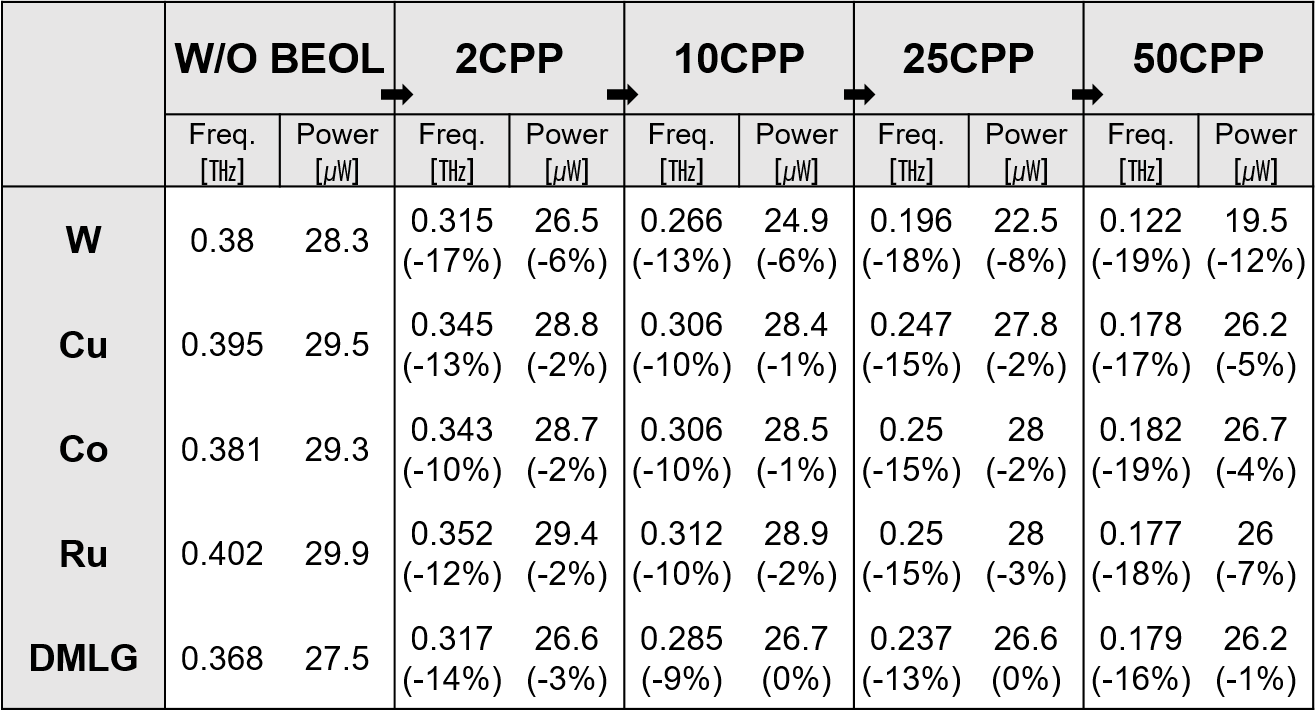##### Table 3. Power, speed value and relative variation at VDD=0.7 V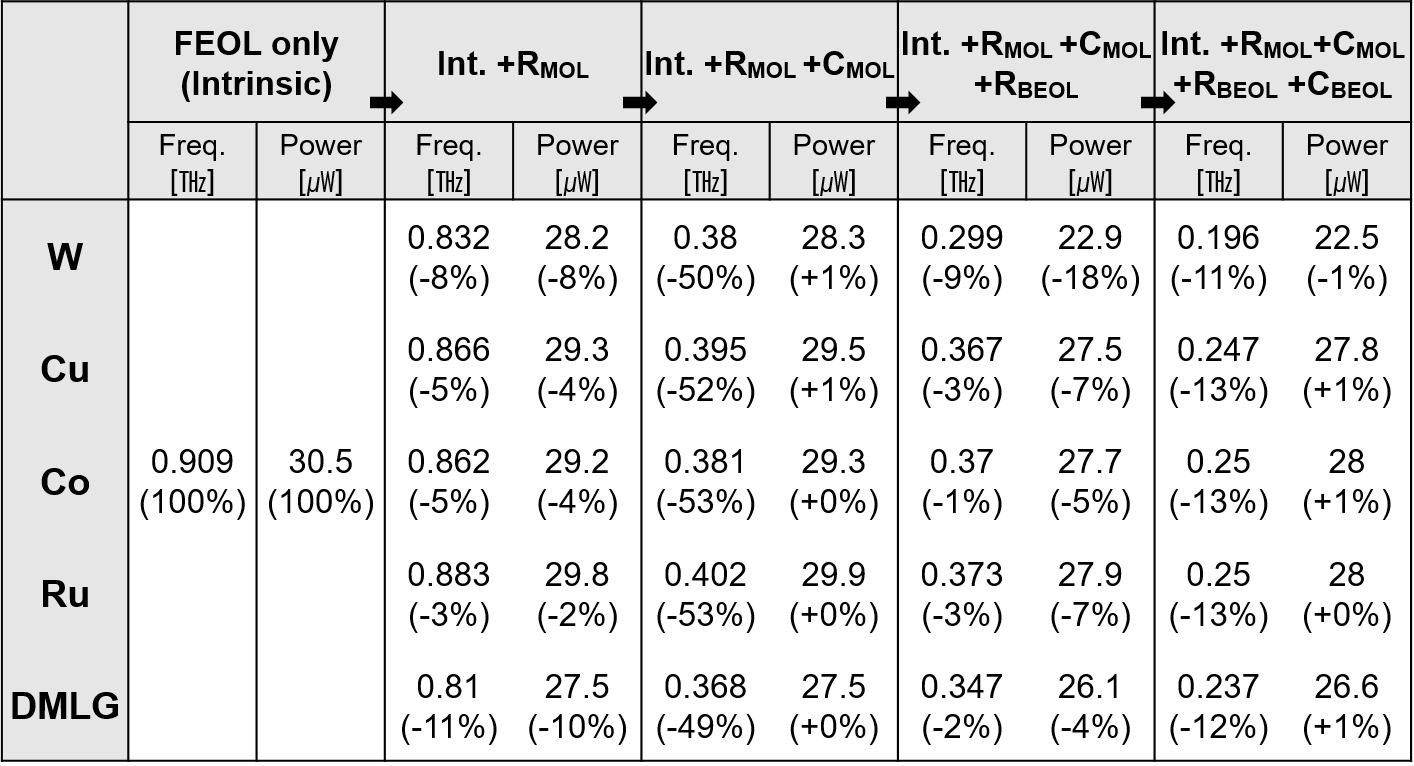##### Table 4. Power, speed value and relative variation at VDD =0.7 V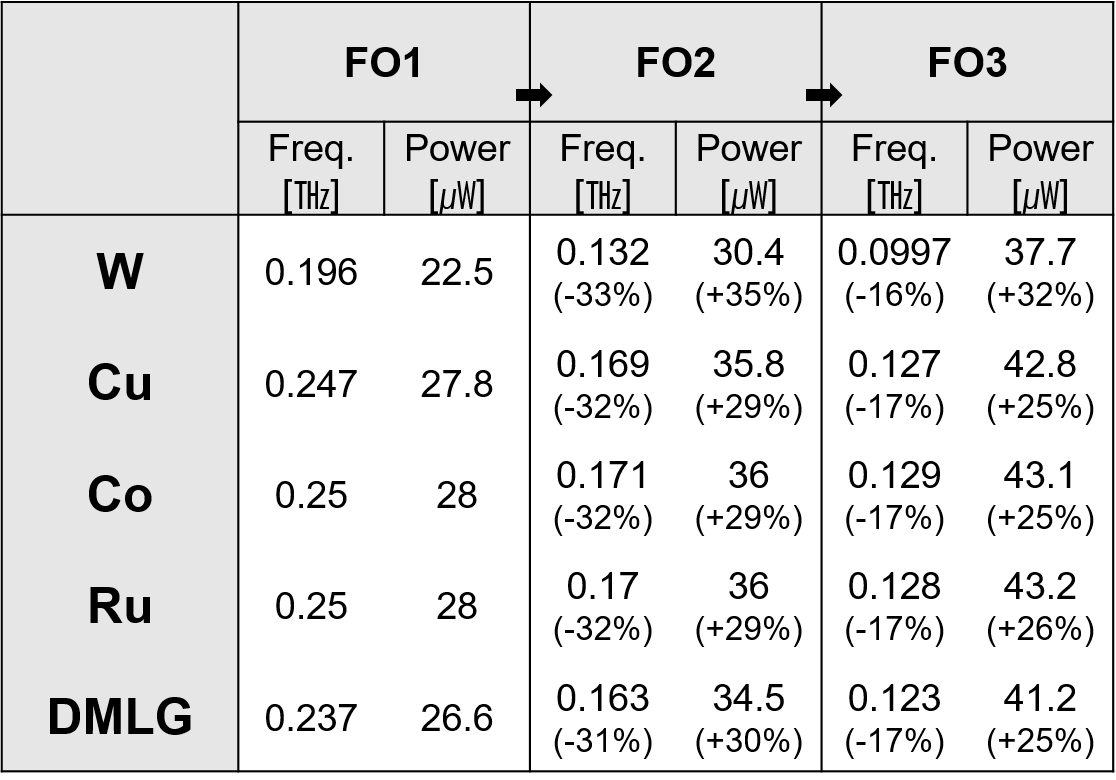##### Table 5. Parameter for SDT/LISD(left) and LIG(right)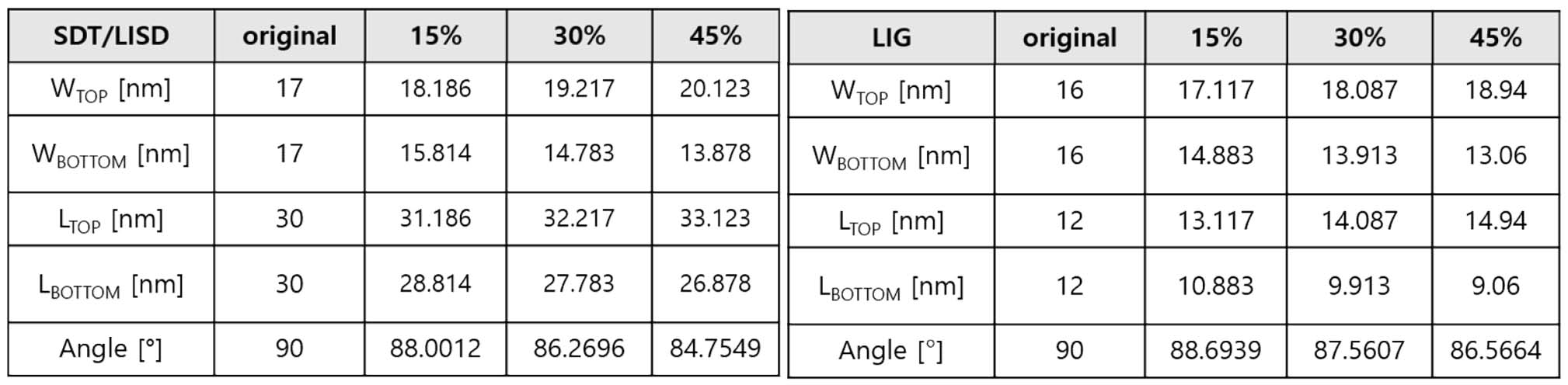##### Table 6. The power, speed value and fluctuation when V$_{\mathrm{DD}}$=0.7~V inFig. 10(a)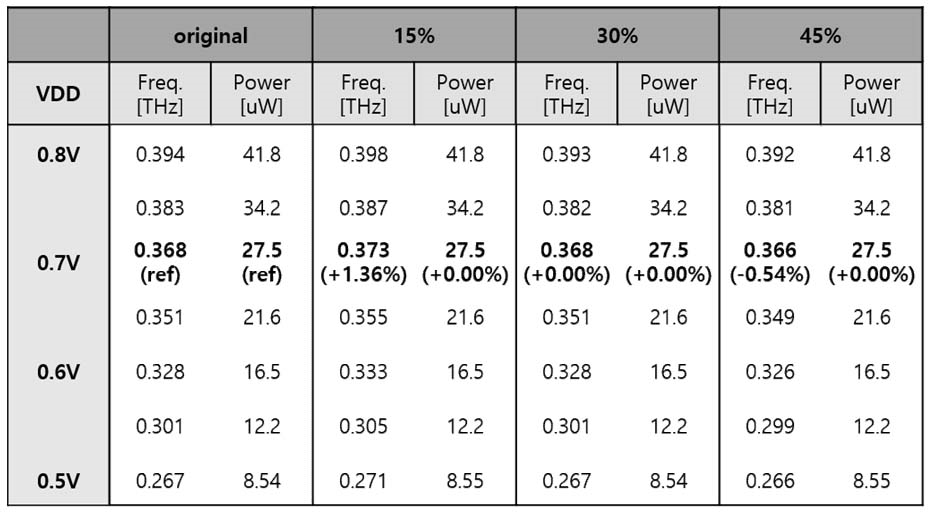##### Table 7. The power, speed value and fluctuation when V$_{\mathrm{DD}}$=0.7~V inFig. 10(b).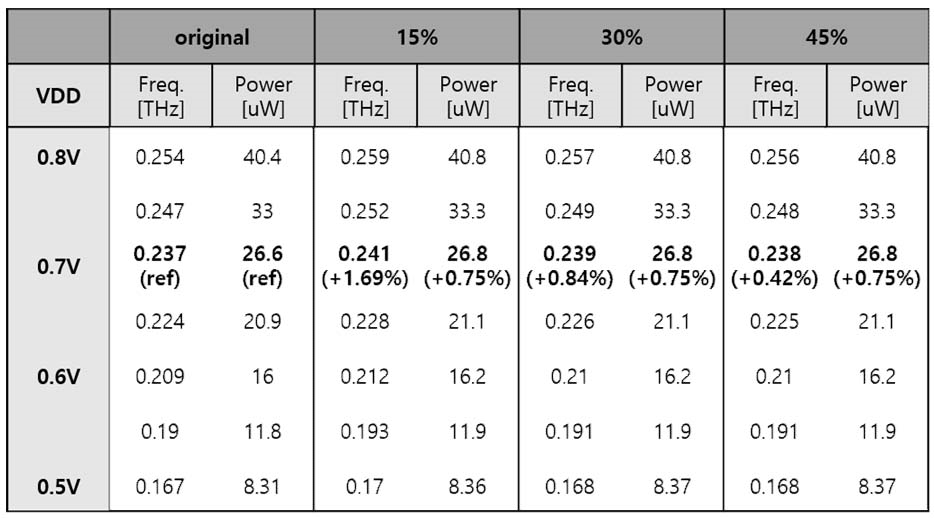##### Fig. 6. (a)~(e) Power versus frequency characteristics for metal option when V$_{\mathrm{DD}}$=0.5 V to 0.8 V is applied after applying W, Cu, Co, Ru and DMLG option to FO1 9-stages INV RO circuit with 3 nm GAA mNS-FET element; (f) FEOL/MOL/BEOL region effective resistance.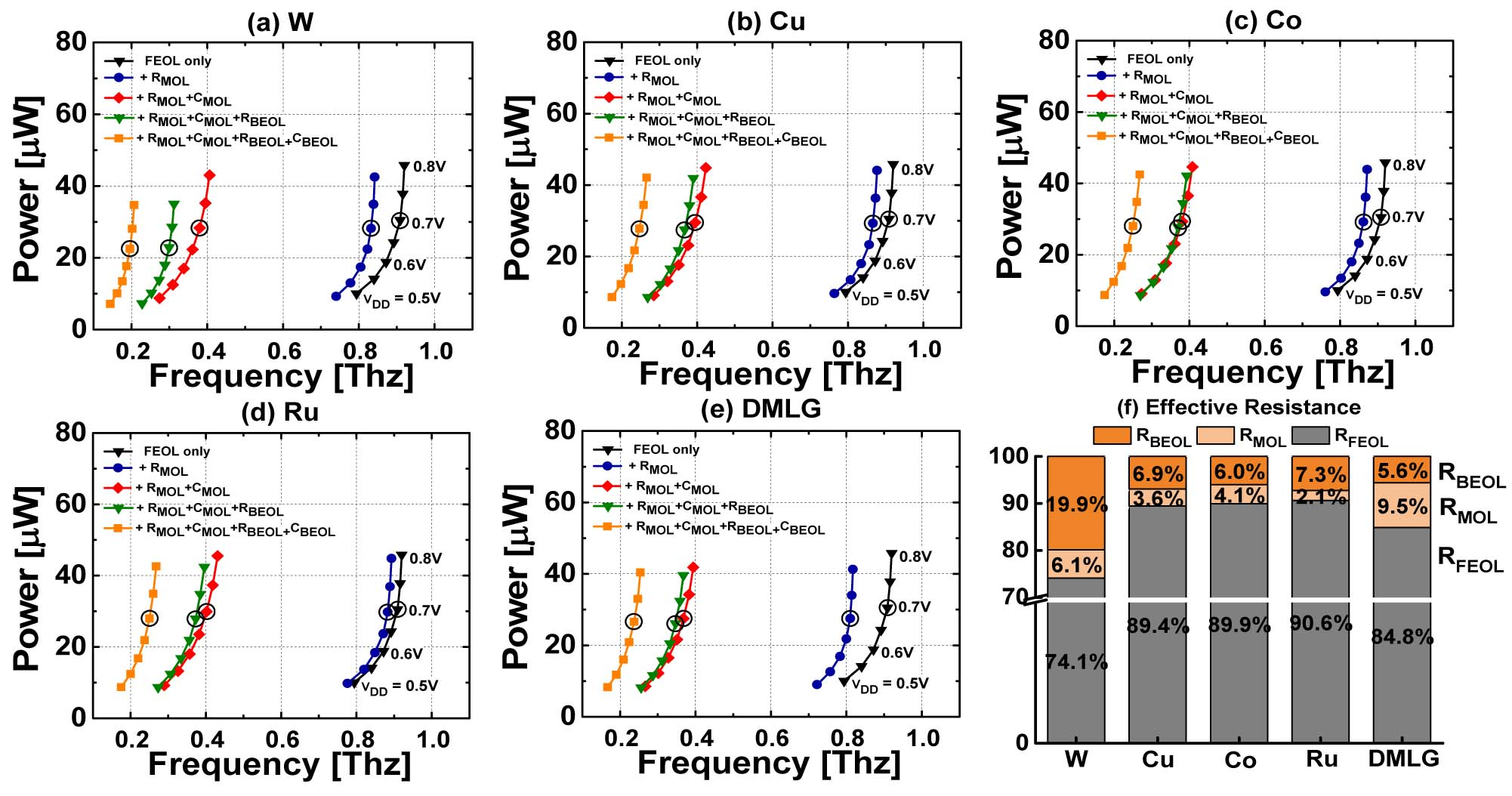##### Fig. 7. (a)~(e) Power versus frequency characteristics for wire length when V$_{\mathrm{DD}}$=0.5 V to 0.8 V is applied after applying W, Cu, Co, Ru, and DMLG option to FO1 9-stages INV RO circuit with 3 nm GAA mNS-FET element; (f) Graph of relative variation, a bar graph means relative power and a line graph means relative frequency(speed).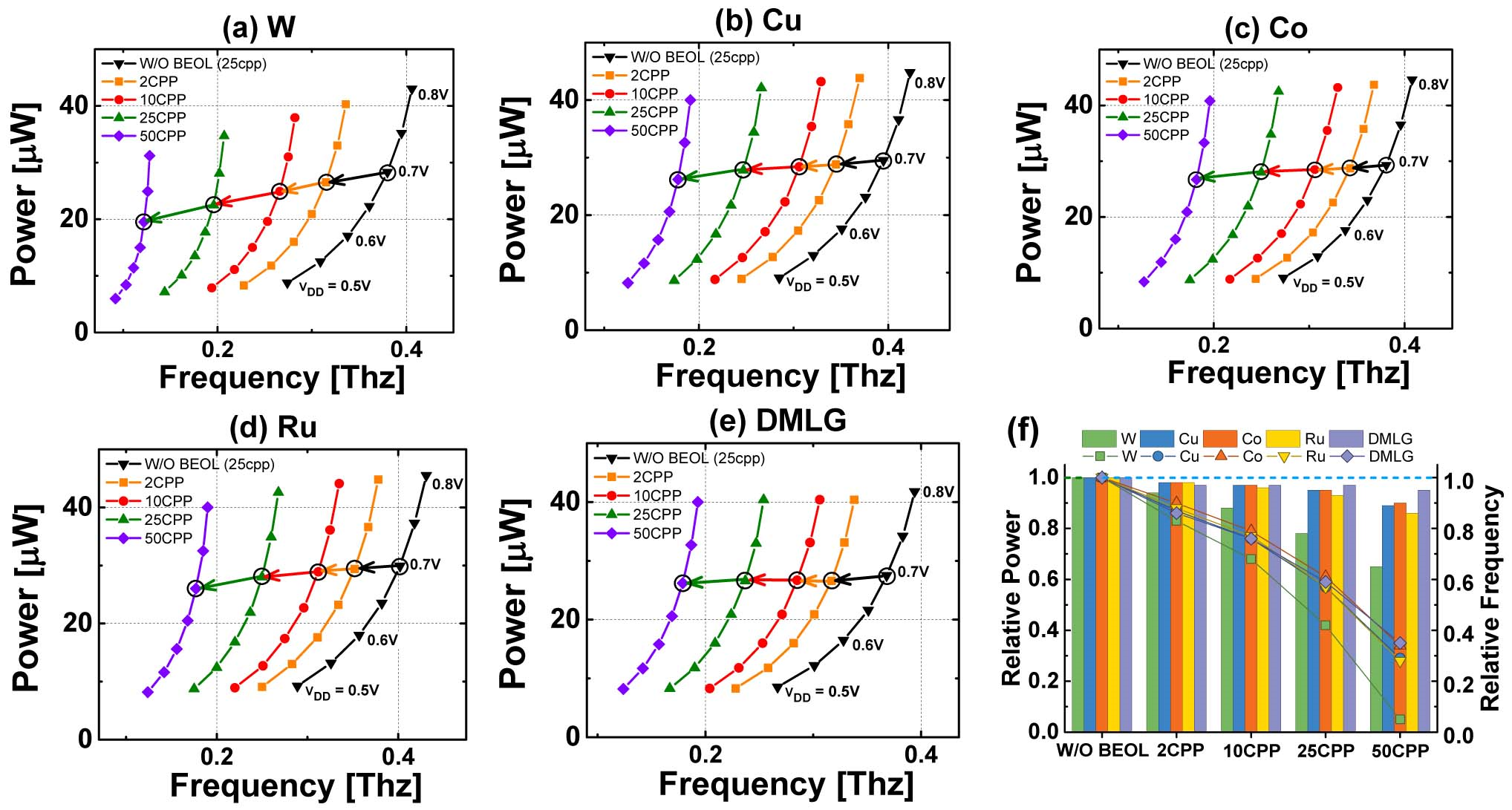##### Fig. 8. (a)~(e) Power versus frequency characteristics for FO number when V$_{\mathrm{DD}}$=0.5 V to 0.8 V is applied after applying W, Cu, Co, Ru, and DMLG option to FO1 9-stages INV RO circuit with 3 nm GAA mNS-FET element; (f) Graph of relative variation, a bar graph means relative power and a line graph means relative frequency(speed).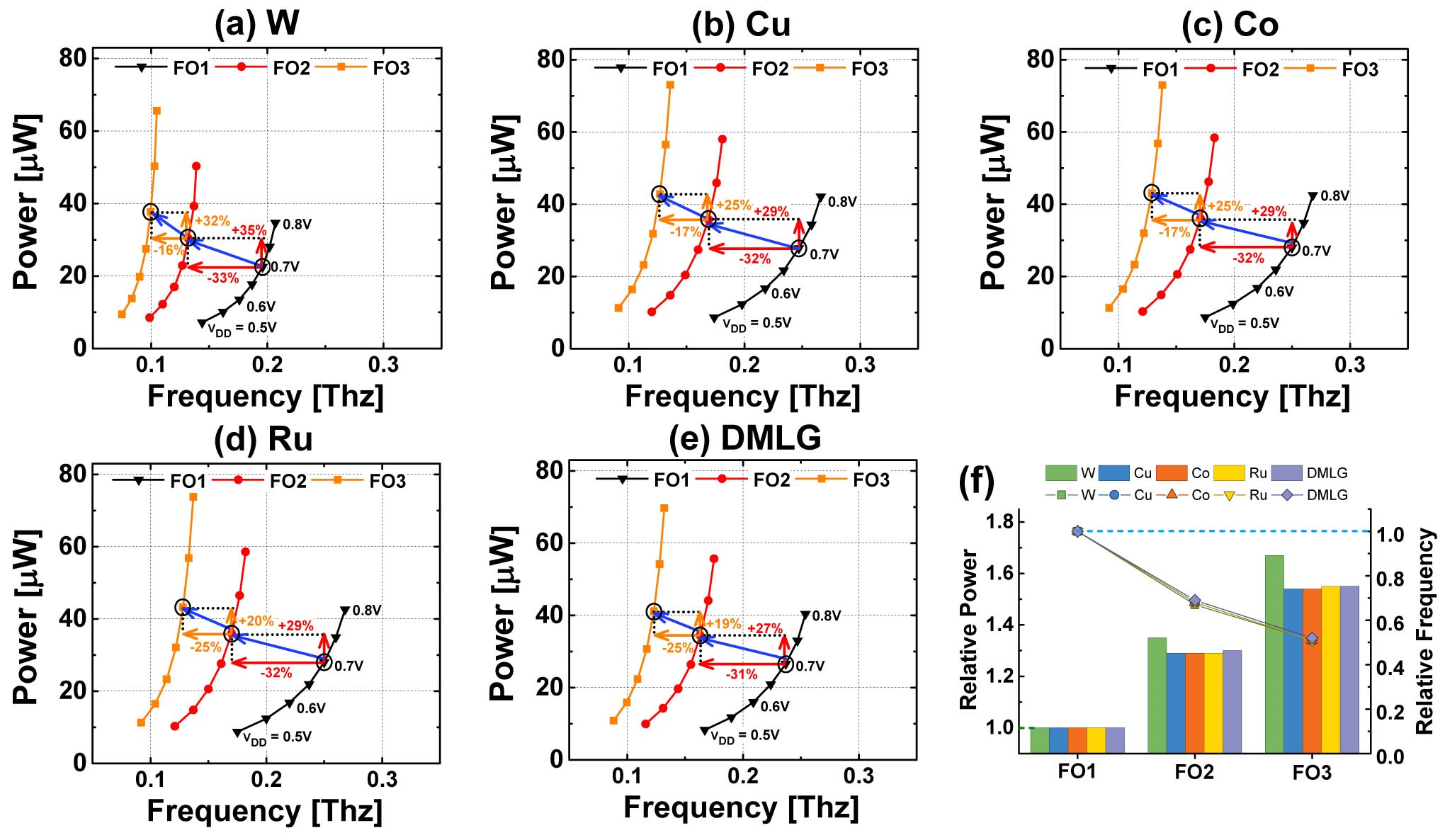##### Fig. 9. (a) SDT/LISD 3D modeling; (b) LIG 3D modeling.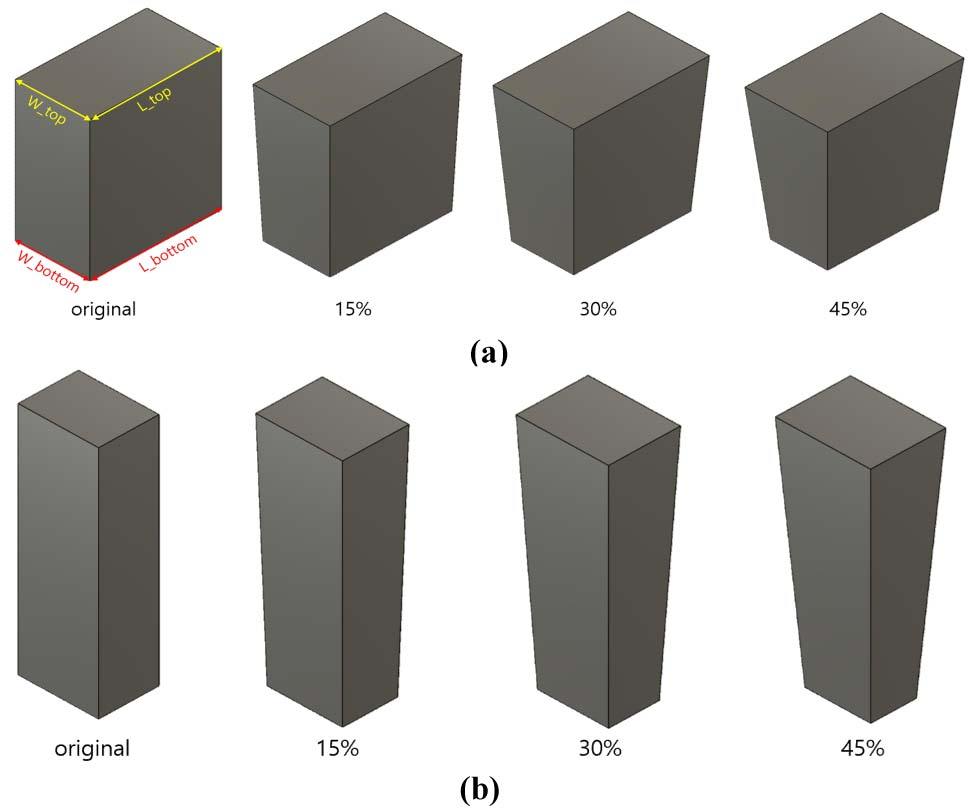##### Fig. 10. Power versus frequency characteristics when (a) the MOL region and (b) the BEOL region is added to intrinsic.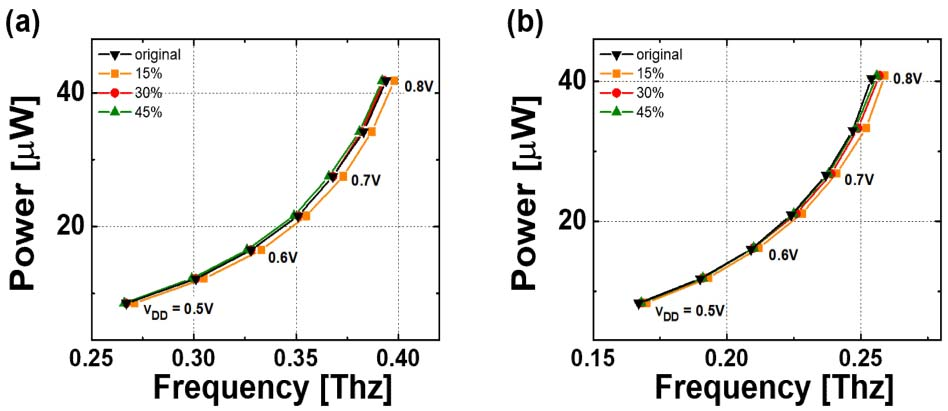##### Fig. 11. (a) Relative frequency of 15%, 30%, and 45% when adding the MOL region with the original power value at V$_{\mathrm{DD}}$=0.7 V; (b) relative power with the original frequency value at V$_{\mathrm{DD}}$=0.7 V.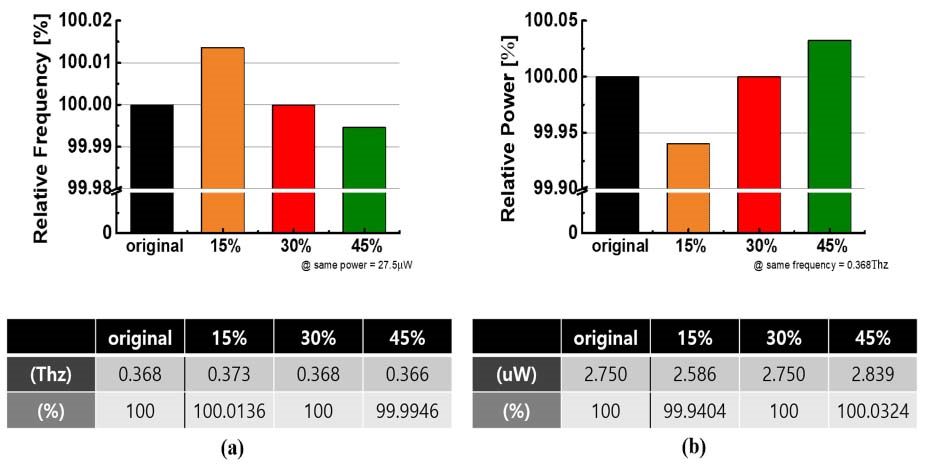##### Fig. 12. (a) Relative frequency of 15%, 30%, and 45% when adding the BEOL region with the original power value at VDD=0.7 V; (b) relative power with the original frequency value at VDD=0.7 V.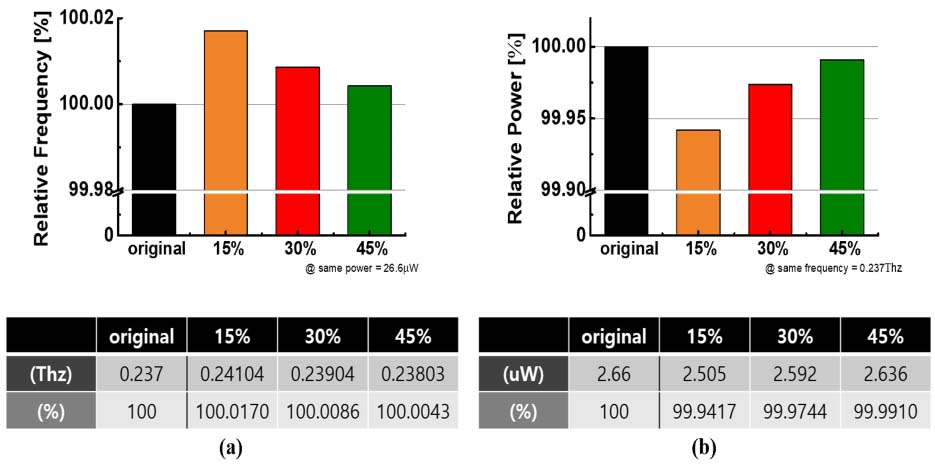## III. CONCLUSIONS

In this work, we analyzed the effect of various wire metal material options on logic circuit properties at 3 nm process node. For this purpose, path-finding PDK was developed by generating a circuit model library of mNS-FET devices expected to be applied at 3 nm process node and applying a wiring model based on five different wire metal materials (W, Cu, Co, Ru, DMLG). Quantitative analysis was performed by applying the developed path-finding PDK to the logic circuit.

The FO1 INV RO circuit was compared with the pre-layout case in which only FEOL was considered, and the circuit characteristics by MOL and BEOL were explored. The speed variations according to the wire metal material decreased by -17%, -8%, -6%, -6%, and -13%, respectively, in W, Cu, Co, Ru, and DMLG, which showed strength in circuit performance in the order of Co = Ru >= Cu > DMLG > W (where, the ‘=’ sign means a small variation of less than 2%)

A summary of circuit characteristics according to the layout scheme (L$_{\mathrm{WIRE}}$, FO numbers) is as follows. L$_{\mathrm{WIRE}}$ is increased from 2CPP to 50CPP length, and the speed variation according to wire load is observed to have better circuit performance in the order of Ru >= Cu >= Co > DMLG >= W in a short wire load(2CPP), but Co >= DMLG >= Cu > W in a long wire load(50CPP). When FO number was increased from 1 to 3 and the speed variation was observed, it was confirmed that the speed was decreased to the same ratio in all wiring metal options compared to FO1. The increase in C$_{\mathrm{EFF}}$ due to FO1 -> FO3 causes an increase in power, where W is +32% and other wire metal options (Cu, Co, Ru, DMLG) are all +25%, which means that W wiring metal degrades the circuit characteristics most in terms of speed and power as FO increases.

These benchmarking results of various wire metal option at a circuit level will be helpful in selecting the suitable interconnect metal for applications that require different circuit speeds and powers at ultra-scaled process nodes in the future.

## ACKNOWLEDGMENTS

Authors are thankful to IC Design Education Center (IDEC) for EDA tool supports. This work was supported by the National Foundation of Korea (NRF) grant funded by the Korea government (MSIT) (No. 2022M3F3A2 A01072215), and partly by the National Foundation of Korea(NRF) grant funded by the Korea government (MSIT) (2021M3F3A2A03017693).

※ MSIT : Ministry of Science and ICT

## References

1
Nagy, Daniel, et al. "FinFET versus gate-all-around nanowire FET: performance, scaling, and variability." IEEE Journal of the Electron Devices Society 6 (2018): 332-340.2
Das, Uttam Kumar, and Tarun Kanti Bhattacharyya. "Opportunities in device scaling for 3-nm node and beyond: FinFET versus GAA-FET versus UFET." IEEE Transactions on Electron Devices 67.6 (2020): 2633-2638..3
Razavieh, Ali, Peter Zeitzoff, and Edward J. Nowak. "Challenges and limitations of CMOS scaling for FinFET and beyond architectures." IEEE Transactions on Nanotechnology 18 (2019): 999-1004.4
Loubet, N., et al. "Stacked nanosheet gate-all-around transistor to enable scaling beyond FinFET." 2017 Symposium on VLSI Technology. IEEE, 2017.5
Bae, Geumjong, et al. "3nm GAA technology featuring multi-bridge-channel FET for low power and high performance applications." 2018 IEEE International Electron Devices Meeting (IEDM). IEEE, 2018.6
Yakimets, D., et al. "Power aware FinFET and lateral nanosheet FET targeting for 3nm CMOS technology." 2017 IEEE International Electron Devices Meeting (IEDM). IEEE, 2017.7
Yang, Giyoung, et al. "Standard Cell Design Optimization with Advanced MOL Technology in 3nm GAA Process." 2022 IEEE Symposium on VLSI Technology and Circuits (VLSI Technology and Circuits). IEEE, 2022.8
Pal, Ashish, et al. "Impact of MOL/BEOL air-spacer on parasitic capacitance and circuit performance at 3 nm node." 2019 International Conference on Simulation of Semiconductor Processes and Devices (SISPAD). IEEE, 2019.9
S. C. Song, "Design/System Technology Co-Optimization for 3nm Node and Beyond." 2021 International Symposium on VLSI Technology, Systems and Applications (VLSI-TSA). IEEE, 2021..10
Li, Hong, et al. "Carbon nanomaterials for next-generation interconnects and passives: Physics, status, and prospects." IEEE Transactions on electron devices 56.9 (2009): 1799-1821.11
Raychowdhury, Arijit, and Kaushik Roy. "Modeling of metallic carbon-nanotube interconnects for circuit simulations and a comparison with Cu interconnects for scaled technologies." IEEE Transactions on Computer-Aided Design of Integrated Circuits and Systems 25.1 (2005): 58-65.12
Zhou, Changjian, and Cary Y. Yang. "3D Nanocarbon Interconnects." 2020 IEEE 15th International Conference on Solid-State & Integrated Circuit Technology (ICSICT). IEEE, 2020.13
Nogami, Takeshi. "Overview of interconnect technology for 7nm node and beyond-New materials and technologies to extend Cu and to enable alternative conductors." 2019 Electron Devices Technology and Manufacturing Conference (EDTM). IEEE, 2019.14
Nogami, Takeshi, et al. "Advanced BEOL Materials, Processes, and Integration to Reduce Line Resistance of Damascene Cu, Co, and Subtractive Ru Interconnects." 2022 IEEE Symposium on VLSI Technology and Circuits (VLSI Technology and Circuits). IEEE, 2022.15
Van der Veen, Marleen H., et al. "Cobalt bottom-up contact and via prefill enabling advanced logic and DRAM technologies." 2015 IEEE International Interconnect Technology Conference and 2015 IEEE Materials for Advanced Metallization Conference (IITC/MAM). IEEE, 2015.16
Jiang, Junkai, et al. "Intercalation doped multilayer-graphene-nanoribbons for next-generation inter-connects." Nano letters 17.3 (2017): 1482-1488.17
Song, Taejoong, et al. "3nm Gate-All-Around (GAA) Design-Technology Co-Optimization (DTCO) for succeeding PPA by Technology." 2022 IEEE Custom Integrated Circuits Conference (CICC). IEEE, 2022.18
Clark, Lawrence T., et al. "ASAP7: A 7-nm finFET predictive process design kit." Microelectronics Journal 53 (2016): 105-115.19
Vashishtha, Vinay, and Lawrence T. Clark. "ASAP5: A predictive PDK for the 5 nm node." Microelectronics Journal (2022): 105481.20
Bhanushali, Kirti, and W. Rhett Davis. "FreePDK15: An open-source predictive process design kit for 15nm FinFET technology." Proceedings of the 2015 Symposium on International Symposium on Physical Design. 2015.21
Dixit, Hemant, et al. "How thin barrier metal can be used to prevent Co diffusion in the modern integrated circuits?." Journal of Physics D: Applied Physics 50.45 (2017): 455103.22
Lee, Yeji, et al. "Investigation on the Effects of Interconnect RC in 3nm Technology Node Using Path-Finding Process Design Kit." IEEE Access 10 (2022): 80695-80702.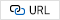23
Agashiwala, Kunjesh, et al. "Demonstration of CMOS-compatible multi-level graphene inter-connects with metal vias." IEEE Transactions on Electron Devices 68.4 (2021): 2083-2091.24
International Roadmap for Devices and Systems (IRDS) 2022 Edition, IEEE-SA, Manhattan, NY, USA, 2022. [Online]. Available : https://irds.ieee. org/editions/202225
Duarte, Juan P., et al. "BSIM-CMG: Standard FinFET compact model for advanced circuit design." ESSCIRC Conference 2015-41st European Solid-State Circuits Conference (ESSCIRC). IEEE, 2015.26
Jiang, Junkai, Jae Hwan Chu, and Kaustav Banerjee. "CMOS-compatible doped-multilayer-graphene interconnects for next-generation VLSI." 2018 IEEE International Electron Devices Meeting (IEDM). IEEE, 2018.27
Cao, Wei, et al. "2-D layered materials for next-generation electronics: Opportunities and challenges." IEEE Transactions on Electron Devices 65.10 (2018): 4109-4121.28
Agashiwala, Kunjesh, et al. "Reliability and performance of CMOS-compatible multi-level graphene interconnects incorporating vias." 2020 IEEE International Electron Devices Meeting (IEDM). IEEE, 2020.Moon Jeong Choi received the B.S. degree in electrical and electronic engineering from Konkuk University, Seoul, Republic of Korea, in 2023. From 2021 to 2022, She was a Research Assistant with the Device Research Laboratory, Seoul. Her research interest includes the development of process design kit.

Juhwan Park received the B.S. degree in electrical and electronic engineering from Konkuk University, Seoul, Republic of Korea, in 2022. He is currently pursuing the M.S. degree in School of Electronic and Electrical Engineering, Sungkyun-kwan University. His research interests include the development of process design kit(PDK) for next generation semiconductor technology.

Seoung Yeol Choi received the B.S. degree in electrical and electronic engineering from Konkuk University, Seoul, Republic of Korea, in 2023. From 2021 to 2022, he was a Research Assistant with the Device Research Laboratory, Seoul. His research interest includes the development of process design kit.

Kyung Bae Kwon received the B.S. degrees in materials, mechanical and automation engineering from the Yanbian University of Science and Technology, Yanji, China, in 2020. He is currently pursuing the M.S. degree in Department of Electrical and Electronics engineering at Konkuk University, Seoul. His current research interests include power device analysis and process design kit(PDK) development.

Ye Ji Lee received the B.S. degree in electrical and electronic engineering from Konkuk University, Seoul, Republic of Korea, in 2022. From 2020 to 2022, she was a Research Assistant with the Device Research Laboratory, Seoul. Her research interest includes the development of process design kit.

WONYEONG JANG received the B.S. degree in electrical and electronic engineering from Konkuk University, Seoul, Republic of Korea, in 2022. From 2020 to 2022, he was a Research Assistant with the Device Research Laboratory, Seoul. His research interest includes the development of process design kit.

Jong Wook Jeon received the B.S. degree in electrical engineering from Sungkyunkwan University, in 2004, and the Ph.D. degree in electrical engineering from Seoul National University, Seoul, South Korea, in 2009. He was a Senior Engineer and a Principal Engineer with the Samsung Research and Development Center, South Korea, from 2009 to 2017. Since 2017, he has been an assistant professor and an associate professor with the department of electrical and electronics engineering, Konkuk University, Seoul, Republic of Korea. His research interest includes design-technology co-optimization (DTCO) of next generation semiconductor technology.